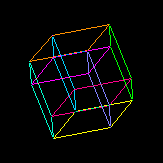# 5-Dim General Relativity (Paul Wesson)Our group is using an extension of general relativity from four to five dimensions where the extra dimension is interpreted as giving rise to matter. Hence the name, Space-Time-Matter theory. Mathematically, STM theory is based on an old embedding theorem of Campbell, which ensures that the 5D Ricci-flat field equations contain the 4D Einstein field equations with matter. Physically, the forms of 4D…

### 5-Dim General Relativity Paul Wesson

5-Dim General Relativity. Our group is using an extension of general relativity from four to five dimensions where the extra dimension is interpreted as giving rise to matter. Hence the name, Space-Time-Matter theory. Mathematically, STM theory is based on an old embedding theorem of Campbell, which ensures that the 5D Ricci-flat field equations contain the 4D Einstein field equations with matter. Physically, the forms of 4D matter are determined by the dependence of the 5D metric on the extra coordinate. Vacuum is especially simple, being the result of a 5D metric whose 4D part is quadratically factorized by the extra coordinate. (There are many papers on this so-called canonical metric, a recent one with references to others being: P.S. Wesson, Phys. Lett. B 722, 1, 2013.) Space-Time-Matter theory, like Membrane theory, goes back to general relativity in the appropriate limit, and is in agreement with all available data. Work on new aspects of the theory, and possible future tests, is carried out by a group of about a dozen people. Our website is 5Dstm.org.

* Late Professor, University of Waterloo, CanadaA projection of the 4-dim hypercube.